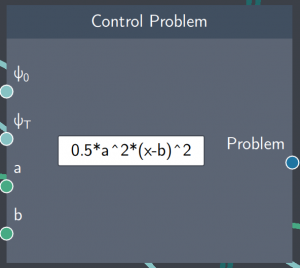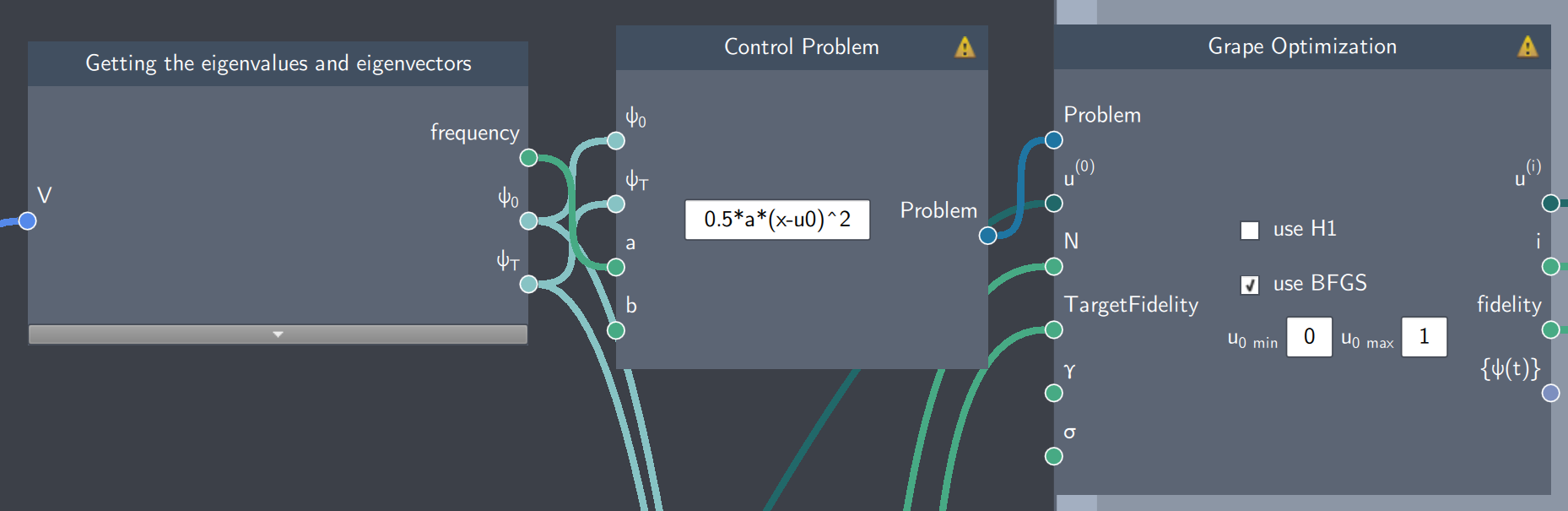# Control Problem

## Description

The Control Problem node lets you define single-particle quantum optimal control problems.## Inputs

The node has the following inputs:

• Initial state ($\psi_{o}$): The initial state of the control problem
• Target state ($\psi_{T}$): The target state of the control problem
• Scalars (a, b): Any scalar values that parameterize the potential or/and the control problem

## Content

The content field of the node consists of an analytical expression that parameterizes a potential  by a control function.

## Output:

• Problem: This node outputs the control problem which is then used as an input for optimization.

## Example

In the example below, a state transfer problem is simulated where a single particle (trapped in a harmonic oscillator potential) is transported from one location to another. The state transfer between an initial and target state is carried out by controlling the potential. In the Control Problem node, the scalar $a$ refers to the frequency of the potential and $u0$  refers to controlling the potential between the two locations. To look at the details of this along with the flowscene, you can look at the single-particle transport exercise.